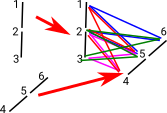Join, Star and Link are important operations on simplectical complexes. This page discusses a FriCAS implementation of these.

## Join

The code includes a join operation like this:

 `simplicialJoin : (a : %, b : %) -> %`

The simplectial join of A and B is a simplectical complex with the vertices and simplexes defined as follows:

##### Vertices

Are disjoint union of vertices of A and vertices of B

vertexSet(simplicialJoin(A,B)) = vertexSet(A)vertexSet(B)

If A and B have common vertices then the vertices of B will be renamed to make then different from A.

##### simplexes

A subset of AB is a simplex of simplicialJoin(A,B) if and only if it is:

• a simplex of A or
• a simplex of B or
• the union of a simplex of A and a simplex of B

#### Properties of simplicialJoin

May be geometrically thought of as a cone over A with tip of shape B.

simplicialJoin is commutative and associative.

dim(simplicialJoin(A,B)) = dim(A) + dim(B) + 1

### Example 1

 Here is an example of the join of 2 lines: [1, 2] and [3, 4] This gives 4 points and the following simplexes: [1, 2] [3, 4] [1, 2 , 3, 4]but each simplex contains all its sub simplexes so the join is just:

(1, 2, 3, 4)

We can calculate this using FriCAS like this:

Note: the implementation of simplicialJoin refactors the indices of the second operand, if necessary, to make sure the points being joined are disjoint.

 ```(1) -> line := line()\$SimplicialComplexFactory(Integer) (1) points 1..2 (1,2) Type: FiniteSimplicialComplex(Integer) (2) -> simplicialJoin(line,line,true) (2) points 1..4 (1,2,3,4) Type: FiniteSimplicialComplex(Integer)```
 The above example is just one simplex so here is an example where the operands have multiple simplexes.Each operand has two simplexes. The result has all 4 combinations of these simplexes.

 ```(3) -> ASIMP := FiniteSimplicialComplex(Integer) (3) FiniteSimplicialComplex(Integer) Type: Type (4) -> v1:List(List(NNI)) := [[1::NNI,2::NNI],[2::NNI,3::NNI]] (4) [[1,2],[2,3]] Type: List(List(NonNegativeInteger)) (5) -> sc1 := simplicialComplex([],v1)\$ASIMP (5) points 1..3 (1,2) (2,3) Type: FiniteSimplicialComplex(Integer) (6) -> simplicialJoin(sc1,sc1,true) (6) points 1..6 (1,2,4,5) (1,2,5,6) (2,3,4,5) (2,3,5,6) Type: FiniteSimplicialComplex(Integer)```

## Star

We can calculate this using FriCAS like this:

 `x`

We can calculate this using FriCAS like this:

 `x`